Next: Formal description Up: Introduction Previous: Introduction   Contents

# Motivations

We find very often in the industry simulators of huge chemical reactors, simulators of huge turbo-compressors, simulators of the path of a satellite in low orbit around earth,... These simulators were written to allow the design engineer to correctly estimate the consequences of the adjustment of one (or many) design variables (or parameters of the problem). Such codes very often demands a great deal of computing power. One run of the simulator can take as much as one or two hours to finish. Some extreme simulations take a day to complete.

These kinds of code can be used to optimize in batch'' the design variables: The research engineer can aggregate the results of the simulation in one unique number which represents the goodness'' of the current design. This final numbercan be seen as the result of the evaluation of an objective function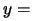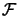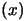where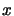is the vector of design variables andis the simulator. We can run an optimization program which find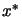, the optimum of.

Most optimization algorithms require the derivatives ofto be available. Unfortunately, we usually don't have them. Very often, there is also some noises ondue to rounding errors. To overcome these limitations, I present here a new optimizer called CONDOR''.

Here are the assumptions needed to use this new optimizer:
• The dimension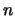of the search space must be lower than 50. For larger dimension the time consumed by this algorithm will be so long and the number of function evaluations will be so huge that I don't advice you to use it.
• No derivatives ofare required. However, the algorithm assumes that they exists. If the function is not continuous, the algorithm can still converge but in a greater time.
• The algorithm tries to minimize the number of evaluations of, at the cost of a huge amount of routine work that occurs during the decision of the next value ofto try. Therefore, the algorithm is particularly well suited for high computing load objective function.
• The algorithm will only find a local minimum of.
• There can be a limited noise on the evaluation of.
• All the design variables must be continuous.
• The non-linear constraints are cheap'' to evaluate.Next: Formal description Up: Introduction Previous: Introduction   Contents
Frank Vanden Berghen 2004-04-19